# Analysing 1.4 billion rows with pythonAnalysing 1.4 billion rows with python . Using pytubes, numpy and matplotlib

The Google Ngram viewer is a fun/useful tool that uses Google’s vast trove of data scanned from books to plot word usage over time. Take, for example, the word Python (case sensitive):

It’s powered from google’s n-gram dataset, a log of the number of times a particular word or sequence of words was spotted by google books during each publication year. While not complete (it doesn’t include every book ever published!), there are millions of books in the set, including books published in the 1500s and up to 2008. The dataset can be freely downloaded here.

I decided to see how easy it would be to reproduce the above graph using Python and my new data loading library: PyTubes

#### Challenges

The 1-gram dataset expands to 27 Gb on disk which is quite a sizable quantity of data to read into python. As one lump, Python can handle gigabytes of data easily, but once that data is destructured and processed, things get a lot slower and less memory efficient.

In total, there are 1.4 billion rows (1,430,727,243) spread over 38 source files, totalling 24 million (24,359,460) words (and POS tagged words, see below), counted between the years 1505 and 2008.

When dealing with 1 billion rows, things can get slow, quickly. And native Python isn’t optimized for this sort of processing. Fortunately numpy is really great at handling large quantities of numeric data. With some simple tricks, we can use numpy to make this analysis feasible.

Handling strings in python/numpy is complicated. The memory overheads of strings in python are quite significant, and numpy only really deals with strings if the length of the string is known and fixed. In this situation, most of the words have different lengths, so that isn’t ideal.

All of the code/examples below were run on a 2016 Macbook Pro with 8 GB ram. Hardware/cloud instances with decent quantities of ram should perform much better

The 1-gram counts are provided as a set of tab-separated files that look like this:

``````Python 1587 4 2
Python 1621 1 1
Python 1651 2 2
Python 1659 1 1``````

Where each row has the following fields:

``````1. Word
2. Year of Publication
3. Total number of times the word was seen
4. Total number of books containing the word``````

To generate the requested graph, we only really need to know some of this information, namely:

``````1. Is the word the one we’re interested in?
2. Year of publication
3. Total number of times the word was seen``````

By just extracting this information, the overheads of handling variable length string data have been avoided, but we still need to compare string values to identify which rows are about the field we’re interested in. This is where pytubes comes in:

``````import tubes

WORD = "Python"

# Set up the data load pipeline
one_grams_tube = (tubes.Each(FILES)
.split()
.multi(lambda row: (
row.get(0).equals(WORD.encode('utf-8')),
row.get(1).to(int),
row.get(2).to(int)
))
)

# Load the data into a numpy array.  By setting a roughly-accurate
# estimated_rows count, pytubes optimizes the allocation pattern.
# fields=True here is redundant, but ensures that the returned ndarray
# uses fields, rather than a single multidimentional array
one_grams = one_grams_tube.ndarray(estimated_rows=500_000_000, fields=True)``````

pytubes1.py

About 170 seconds (3 minutes) later, one_grams is a numpy array with ~1.4 billion rows, looking like this (headers added for clarity):

``````═══════════╤════════╤═════════╕
│   Is_Word │   Year │   Count │
╞═══════════╪════════╪═════════╡
│         0 │   1799 │       2 │
├───────────┼────────┼─────────┤
│         0 │   1804 │       1 │
├───────────┼────────┼─────────┤
│         0 │   1805 │       1 │
├───────────┼────────┼─────────┤
│         0 │   1811 │       1 │
├───────────┼────────┼─────────┤
│         0 │   1820 │     ... │
╘═══════════╧════════╧═════════╛``````

From here, it’s just a question of using numpy methods to calculate some things:

#### Total word count for each year

Google shows the % occurrence of each word (number of times a word occurs/total number of words published that year) which is somewhat more useful that just the raw word count. To calculate this, we need to know what the total word count is.

Luckily numpy makes this really simple:

``````last_year = 2008
YEAR_COL = '1'
COUNT_COL = '2'

year_totals, bins = np.histogram(
one_grams[YEAR_COL],
density=False,
range=(0, last_year+1),
bins=last_year + 1,
weights=one_grams[COUNT_COL]
)``````

pytubes2.py

Plotting this shows how many words google has collected for each year: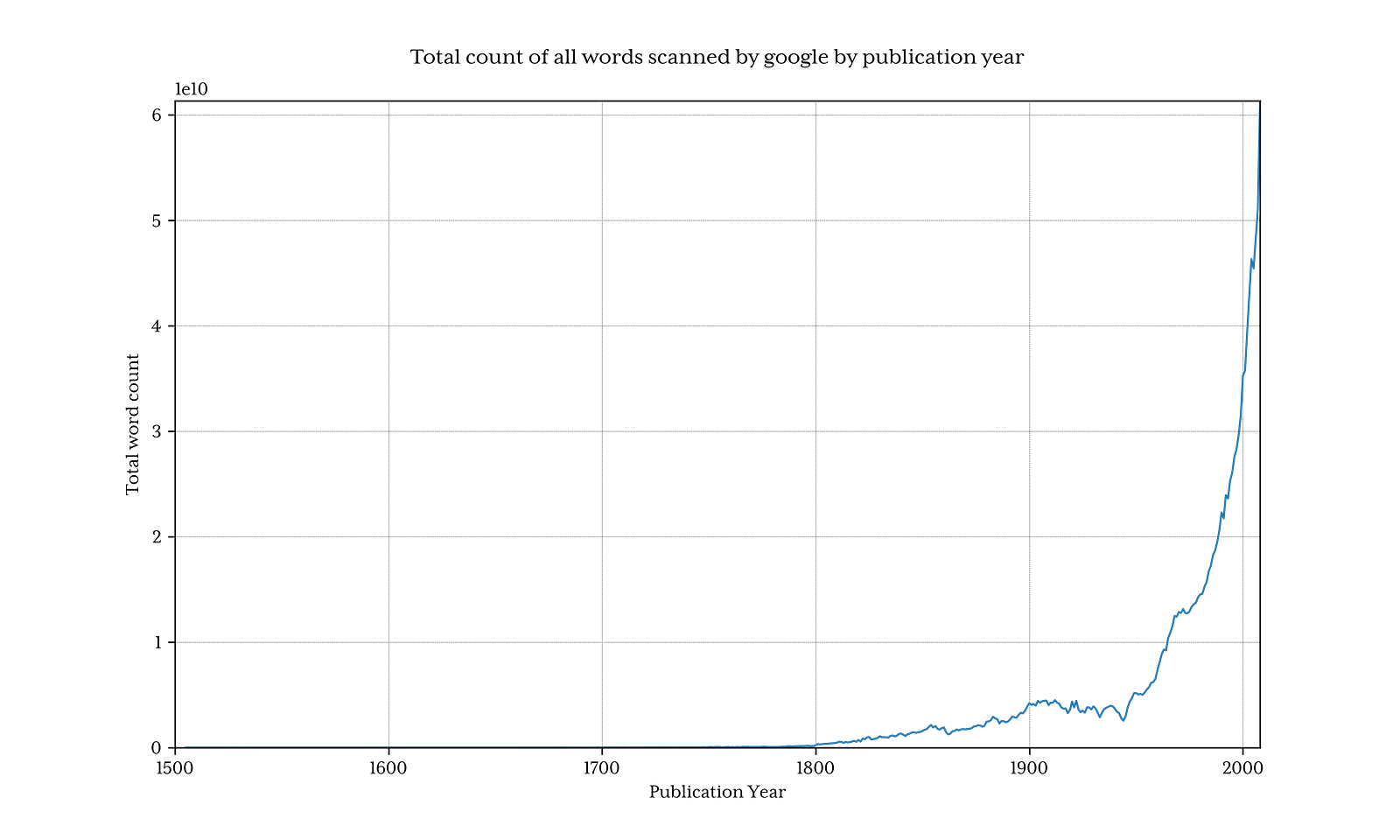What’s clear is that before 1800, the volume of data falls off quickly, and thus can skew results, and hide interesting patterns. To counter this, let’s only include data after 1800:

``````one_grams_tube = (tubes.Each(FILES)
.split()
.skip_unless(lambda row: row.get(1).to(int).gt(1799))
.multi(lambda row: (
row.get(0).equals(word.encode('utf-8')),
row.get(1).to(int),
row.get(2).to(int)
))
)``````

pytubes3.py

Which returns 1.3 Billion rows (only 3.7% of words are recorded from before 1800)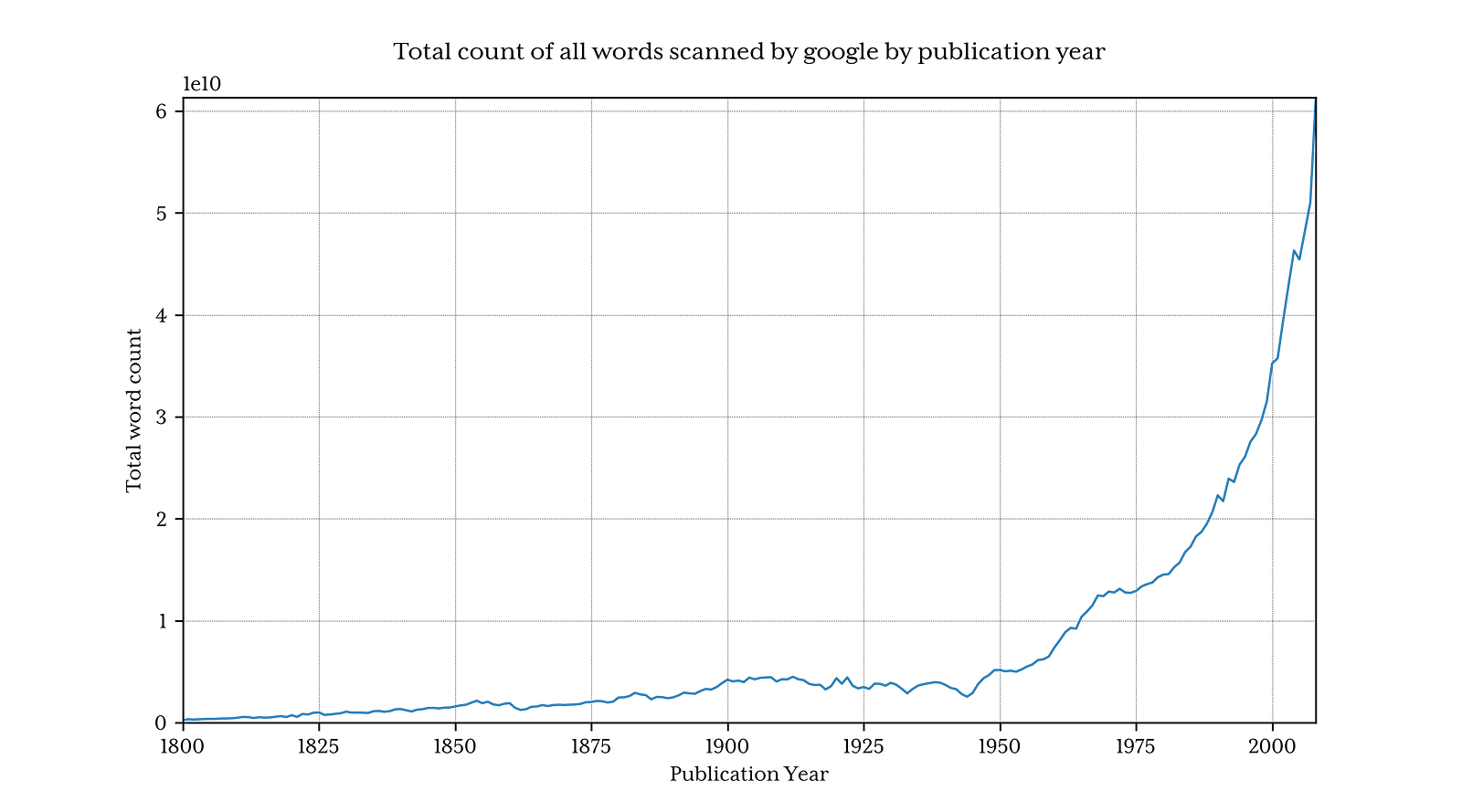#### Python % by Year

Getting the % counts for python is now surprisingly easy.

Using the simple trick of making the year-based arrays 2008 elements long means that the index for each year equals the year number, so finding the entry for, say, 1995 is just a question of getting the 1,995 th element.

It’s not even worth using numpy operations for this:

``````# Find the matching rows (where the first column is True)
word_rows = one_grams[IS_WORD_COL]
# Create an empty array to hold the per-year % values
word_counts = np.zeros(last_year+1)
# Iterate over each matching row (for a matching word, there should just be 1,000s of rows)
for _, year, count in one_grams[word_rows]:
# Set the relevant word_counts row to the calculated value
word_counts[year] += (100*count) / year_totals[year]``````

pytubes4.py

Plotting the resulting word_counts: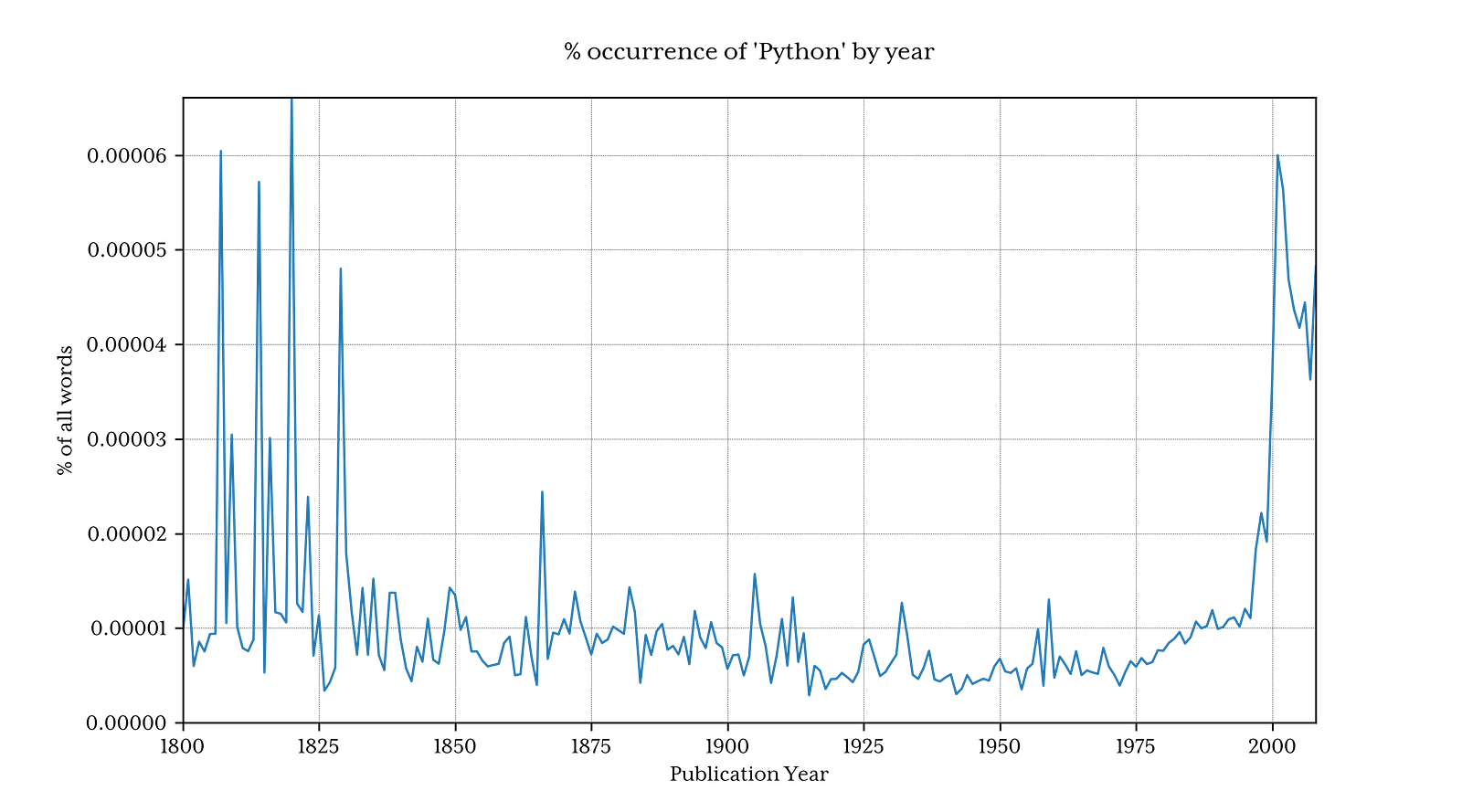which turns out pretty similar in shape to Google’s version:The actual % numbers don’t match up at all, and I think this is because the downloadable dataset includes words tagged with various parts of speech (for example: Python_VERB). This is not explained well in the google page for this dataset, and raises several questions:

• how does one use Python as a verb?
• Do the counts for ‘Python’ include the counts for ‘Python_VERB’? etc.

Luckily, it’s clear that the method I’ve used produces a similar-enough shape of graph as google that the relative trends are not affected, so for this exploration, I’m not going to try to fix that.

#### Performance

Google produces its graph in ~1 second, compared with about 8 minutes with this script, but this is reasonable. The backend to Google’s word count will be working from significantly prepared views of the dataset.

For example, pre-calculating the per-year total word count and storing it in a single lookup table would save significant time here. Likewise, storing the word counts in a single database/file and indexing the first column would eliminate almost all of the processing time.

What this exploration does show, however, is that using numpy and the fledgeling pytubes, it’s possible to load, process, and extract some arbitrary statistical information from raw billion-row datasets in a reasonable time, using standard commodity hardware and Python.

### Language Wars

Just to prove the concept with a slightly more complex example, I decided to compare the relative mention rates of three programming languages: Python, Pascal, and Perl.

The source data is quite noisy (it includes all English words used, not just programming language mentions and, for example, python also has a non-techical meaning!) to try to adjust for this, two things things were done:

1. Only the Title case forms of the names are matched (Python, not python)
2. Each language’s mention count has been be shifted by the mean % count between 1800 and 1960, Given Pascal as a language was first mentioned in 1970, this should give a reasonable baseline.

#### Results: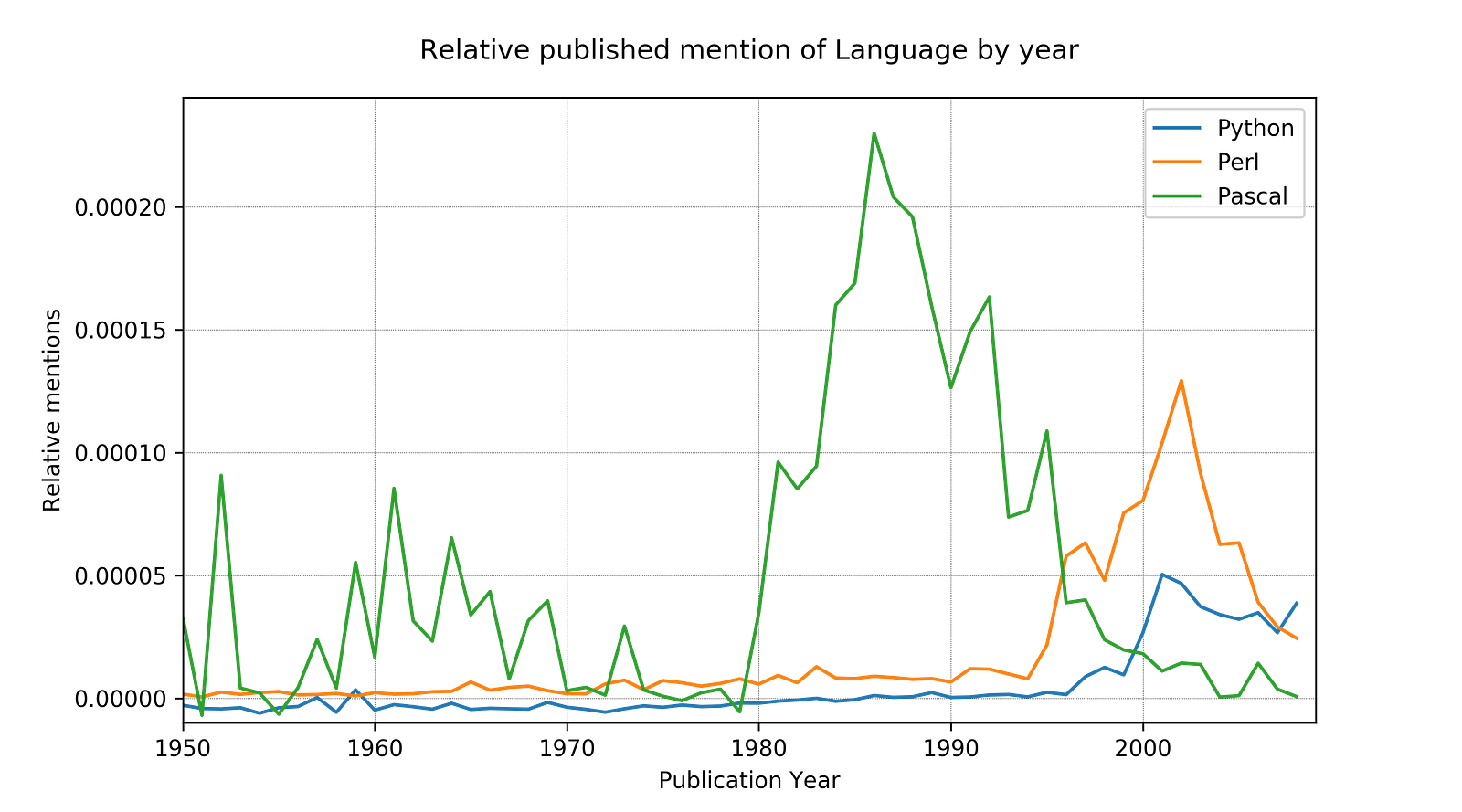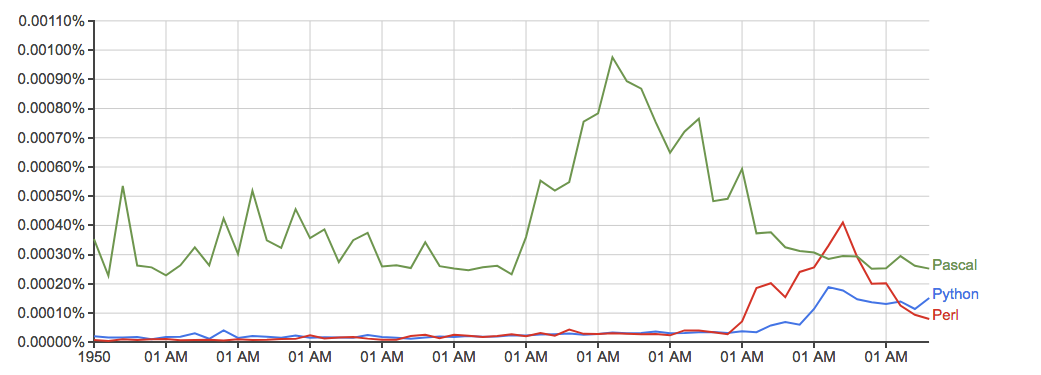Run Time: Just over 10 minutes

#### Future PyTubes Improvements

At the moment, pytubes only has a single concept of an integer, which is a 64-bit int. This means that numpy arrays generated by pytubes use i8 dtypes for all integers. In some cases (like the ngrams data) 8-byte integers are a little over-kill, and waste memory (the full ndarray here is about 38Gb, this could easily be reduced by 60% with better dtypes). I plan to add some level of 1, 2 and 4 byte integer support soon (https://github.com/stestagg/pytubes/issues/9)

More filter logic — the Tube.skip_unless() method is a simple way to filter rows, but lacks any ability to combine conditions (AND/OR/NOT). This will make reducing the volume of loaded data much faster for some use-cases.

Better string matching —Simple tests like: startswith, endswith, contains, and is_one_of are easy to add, and significantly improve the usefulness when loading lots of string data.

## Basic Data Types in Python | Python Web Development For Beginners

In the programming world, Data types play an important role. Each Variable is stored in different data types and responsible for various functions. Python had two different objects, and They are mutable and immutable objects.

## How To Compare Tesla and Ford Company By Using Magic Methods in Python

Magic Methods are the special methods which gives us the ability to access built in syntactical features such as ‘<’, ‘>’, ‘==’, ‘+’ etc.. You must have worked with such methods without knowing them to be as magic methods. Magic methods can be identified with their names which start with __ and ends with __ like __init__, __call__, __str__ etc. These methods are also called Dunder Methods, because of their name starting and ending with Double Underscore (Dunder).

## Python Programming: A Beginner’s Guide

Python is an interpreted, high-level, powerful general-purpose programming language. You may ask, Python’s a snake right? and Why is this programming language named after it?

## Hire Python Developers

Are you looking for experienced, reliable, and qualified Python developers? If yes, you have reached the right place. At **[HourlyDeveloper.io](https://hourlydeveloper.io/ "HourlyDeveloper.io")**, our full-stack Python development services...

## Python any: How to Check If Element is Iterable or Not

Python any() function returns True if any element of an iterable is True otherwise any() function returns False. The syntax is any().